MP Board Class 8th Maths Solutions Chapter 6 Square and Square Roots Ex 6.3

Question 1.
What could be the possible ‘ones’ digits of the square root of each of the following numbers?
(i) 9801
(ii) 99856
(iii) 998001
(iv) 657666025
Solution:
(i) We know that the ‘ones’ place of the square of 1 and 9 is 1.
∴ The possible ‘ones’ digits of the square root of 9801 are 1 and 9.
(ii) We know that the ‘ones’ place of the square of 4 and 6 is 6.
∴ The possible ‘ones’ digits of the square root of 99856 are 4 and 6.
(iii) We know that the ‘ones’ place of the square of 1 and 9 is 1.
∴ The possible ‘ones’ digits of the square root of 998001 are 1 and 9.
(iv) We know that ‘ones’ place of the square of 5 is 5.
∴ The possible ‘ones’ digit of the square root of 657666025 is 5.Question 2.
Without doing any calculation, find the numbers which are surely not perfect squares,
(i) 153
(ii) 257
(iii) 408
(iv) 441
Solution:
We know that the numbers ending with 2, 3, 7 or 8 are not perfect squares. So, (i), (ii) and (iii) are surly not perfect squares.
(iv) Since, the number 441 ends with 1. Thus, 441 may or may not be a perfect square.Question 3.
Find the square root of 100 and 169 by the method of repeated subtraction.
Solution:
First consider 100.
(1) 100 – 1 = 99
(2) 99 – 3 = 96
(3) 96 – 5 = 91
(4) 91 – 7 = 84
(5) 84-9 = 75
(6) 75 – 11 = 64
(7) 64 – 13 = 51
(8) 51 – 15 = 36
(9) 36 – 17 = 19
(10) 19 – 19 = 0.
∴ $$\sqrt{100}$$ = 10.
Now, consider 169
(1) 169 – 1 = 168
(2) 168 – 3 = 165
(3) 165 – 5 = 160
(4) 160 – 7 = 153
(5) 153 – 9 = 144
(6) 144 – 11 = 133
(7) 133 – 13 = 120
(8) 120 – 15 = 105
(9) 105-17 = 88
(10) 88 – 19 = 69
(11) 69 – 21 = 48
(12) 48 – 23 = 25
(13) 25 – 25 = 0.
∴ $$\sqrt{169}$$ = 13.Question 4.
Find the square roots of the following numbers by the Prime Factorisation Method.
(i) 729
(ii) 400
(iii) 1764
(iv) 4096
(v) 7744
(vi) 9604
(vii) 5929
(viii) 9216
(ix) 529
(x) 8100
Solution: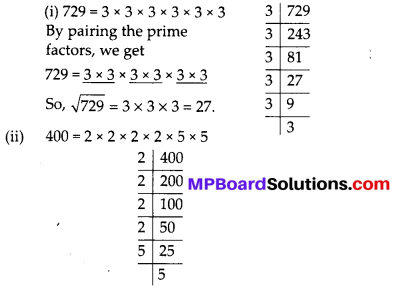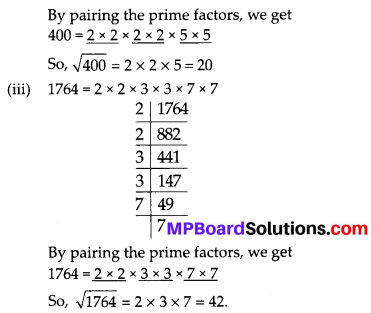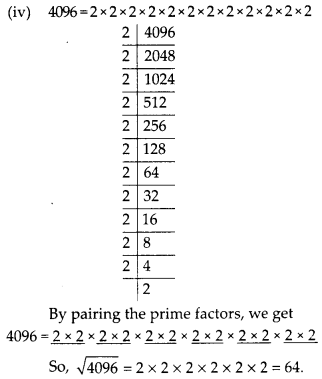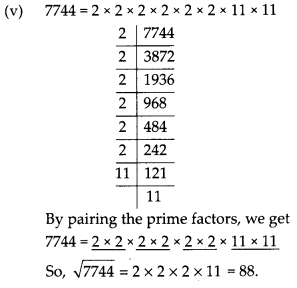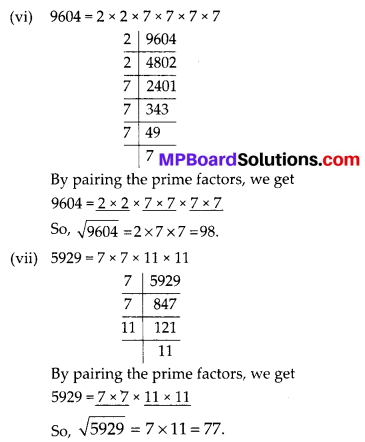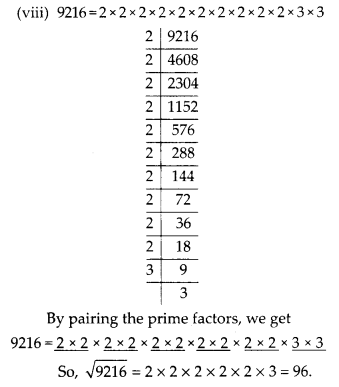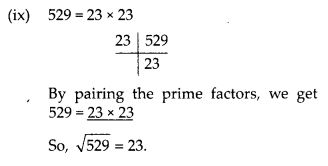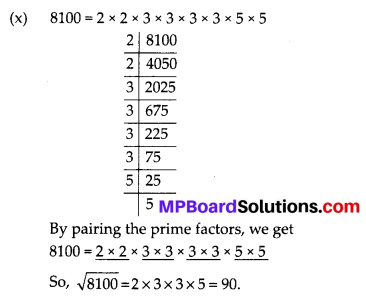Question 5.
For each of the following numbers, find the smallest whole number by which it should be multiplied so as to get a perfect square number. Also find the square root of the square number so obtained.
(i) 252
(ii) 180
(iii) 1008
(iv) 2028
(v) 1458
(vi) 768
Solution:
(i) We have, 252 = 2 × 2 × 3 × 3 × 7
The smallest whole number is 7 by which 252 should be multiplied so as to get a perfect square.
252 × 7 = 2 × 2 × 3 × 3 × 7 × 7
Now each prime factor is in a pair. Therefore, 252 × 7 = 1764 is a perfect square.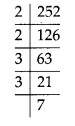(ii) We have, 180 = 2 × 2 × 3 × 3 × 5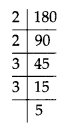The smallest whole number is 5, by which 180 should be multiplied so as to get a perfect square.
180 × 5 = 2 × 2 × 3 × 3 × 5 × 5
So, $$\sqrt{900}$$ = 2 × 3 × 5 = 30.

(iii)
We have,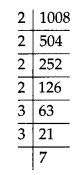1008 = 2 × 2 × 2 × 2 × 3 × 3 × 7
The smallest whole number is 7, by which 1008 should be multiplied so as to get a perfect square.
1008 × 7 = 2 × 2 × 2 × 2 × 3 × 3 × 7 × 7
Now each prime factor is in pair. Therefore,
1008 × 7 = 7056 is a perfect square.
So, $$\sqrt{7056}$$ = 2 × 2 × 3 × 7 = 84.

(iv) We have, 2028 = 2 × 2 × 3 × 13 × 13
The smallest whole number is 3 by which 2028 should be multiplied so as to get a perfect square.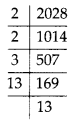2028 × 3 = 2 × 2 × 3 × 3 × 13 × 13
Now each prime factor is in pair. Therefore, 2028 × 3 = 6084 is a perfect square.
So, $$\sqrt{6084}$$ = 2 × 3 × 13 = 78.

(v) We have, 1458 = 2 × 3 × 3 × 3 × 3 × 3 × 3
The smallest whole number is 2, by which 1458 should be multiplied so as to get a perfect square.
1458 × 2 = 2 × 2 × 3 × 3 × 3 × 3 × 3 × 3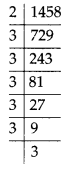Now each prime factor is in pair.
Therefore, 1458 × 2 = 2916 is a perfect square.
So, $$\sqrt{2916}$$ = 2 × 3 × 3 × 3 = 54

(vi) We have, 768 = 2 × 2 × 2 × 2 × 2 × 2 × 2 × 2 × 3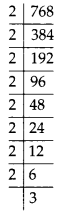The smallest whole number is 3, by which 768 should be multiplied so as to get a perfect square.
768 × 3 = 2 × 2 × 2 × 2 × 2 × 2 × 2 × 2 × 3 × 3
Now each prime factor is in pair.
Therefore, 768 × 3 = 2304 is a perfect square.
So, $$\sqrt{2304}$$ = 2 × 2 × 2 × 2 × 3 = 48.Question 6.
For each of the following numbers, find the smallest whole number by which it should be divided so as to get a perfect square. Also find the square root of the square number so obtained,
(i) 252
(ii) 2925
(iii) 396
(iv) 2645
(v) 2800
(vi) 1620
Solution:
(i) We have 252 = 2 × 2 × 3 × 3 × 7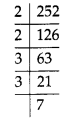We find that 252 should be divided by 7, to get a perfect square.
252 ÷ 7 = 36 = 2 × 2 × 3 × 3
Therefore, the required smallest number is 7.

(ii) We find that 2925 = 3 × 3 × 5 × 5 × 13
We find that 2925 should be divided by 13, to get a perfect square.
2925 ÷ 13 = 225 = 3 × 3 × 5 × 5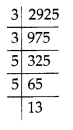Therefore, the required smallest number is 13.
Also, $$\sqrt{225}$$ = 3 × 5 = 15.

(iii) We have, 396 = 2 × 2 × 3 × 3 × 11
We find that 396 should be divided by 11, to get a perfect square.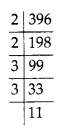396 ÷ 11 = 36 = 2 × 2 × 3 × 3
Therefore, the required smallest number is 11.
Also, $$\sqrt{36}$$ = 2 × 3 = 6.

(iv) We have, 2645 = 5 × 23 × 23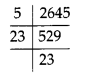We find that 2645 should be divided by 5, to get a perfect square.
2645 ÷ 5 = 529 = 23 × 23
Therefore, the required smallest number is 5.
Also, $$\sqrt{529}$$ = 23.

(v) We have, 2800 = 2 × 2 × 2 × 2 × 5 × 5 × 7
We find that 2800 should be divided by 7, to get a perfect square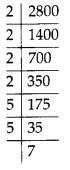2800 ÷ 7 = 400 = 2 × 2 × 2 × 2 × 5 × 5
Therefore, the required smallest number is 7.
Also, $$\sqrt{400}$$ = 2 × 2 × 5 = 20.

(vi) We have,
1620 = 2 × 2 × 3 × 3 × 3 × 3 × 5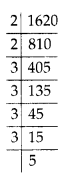Question 7.
The students of Class VIII of a school donated ₹ 2401 in all, for the Prime Minister’s National Relief Fund. Each student donated as many rupees as the number of students in the class. Find the number of students in the class.
Solution:
We have, 2401 = 7 × 7 × 7 × 7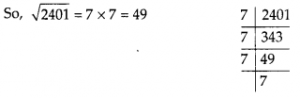We find that the number of students in the class is 49.Question 8.
2025 plants are to be planted in a garden in such a way that each row contains as many plants as the number of rows. Find the number of rows and the number of plants in each row.
Solution:
Total number of plants = 2025
The plants are planted in a garden in such a way that each row contains as many plants as the number of rows.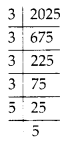2025 = 3 × 3 × 3 × 3 × 5 × 5
∴ Number of plants in each row = $$\sqrt{2025}$$ = 3 × 3 × 5 = 45
So, number of rows = number of plants.
Thus, the number of rows = 45
and number of plants in each row = 45.

Question 9.
Find the smallest square number that is divisible by each of the numbers 4,9 and 10.
Solution:
The smallest number divisible by each 4, 9 and 10 is their LCM.
The LCM of 4, 9 and 10 is 2 × 2 × 3 × 3 × 5 = 180.
Now, prime factorisation of 180 is
180 = 2 × 2 × 3 × 3 × 5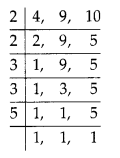In order to get a perfect square, each factor of 180 must be paired.
So, we need to make pair of 5.
∴ 180 should be multiplied by 5.
Hence, the required number is 180 × 5 = 900.Question 10.
Find the smallest square number that is divisible by each of the numbers 8, 15 and 20.
Solution:
The smallest number divisible by each 8, 15 and 20, is their LCM.
The LCM of 8, 15 and 20 is 2 × 2 × 2 × 3 × 5 = 120Now prime factorisation of 120 is
120 = 2 × 2 × 2 × 3 × 5 ….. (i)
In order to get a perfect square, each factor of 120 must be paired.
Thus we multiply (i) by 2 × 3 × 5 = 30, we get 120 × 30 = 3600.